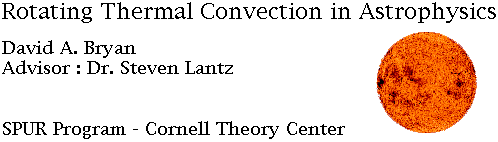## Introduction:

In this project, we worked to construct a simplified mathematical model of certain physical processes within the Sun. Using discrete mathematical methods, and the computing resources of the Cornell Theory Center, we attempted to explore some of the inner workings of the Sun through computer simulation.

Research into this field will allow scientists to better understand how the Sun works, and why the structures we see on the Sun develop in the way they do.

As my part of this project, I wrote several programs to solve portions of the complete differential equations which described our model. FORTRAN was used to write the code, compiled using IBM xlf 3.1, and the code was run on various IBM RS/6000 workstations and the IBM SP-1 and SP-2 supercomputers at the Theory Center.

In addition to FORTRAN, a language called Wave Advantage was used for generation of the mathematical functions used as test input data while creating and debugging the code. In addition, the Wave Advantage software was used for some simple visualization of the results of the initial computations.

For later visualization of results, IBM's Data Explorer was used. This program allowed for greater flexibility in the display and visualization of the data.

## Objective:

In these experiments, we attempted to reconstruct a phenomenon in fluid dynamics known as a thermal Rossby wave, a type of traveling wave. This occurs when two dimensional convective rolls are influenced by rotation.

## Method:

The method for constructing this model was to define a set of differential equations that could match phenomena believed to be occurring inside the Sun. Once these equations are known, we can then use the computer to solve these equations approximately in space and time, observing the results of the time stepping on the arrays that represent the various physical variables of the equations (temperature, pressure, velocity etc.) In each stage the model is simply a set of arrays which represent these physical quantities at discrete points on a regular grid. As more terms of the equations used are added, more complex behavior can be exhibited by the arrays of the model.

For solving these differential equations, several methods were employed. The two methods chosen for our project were the spectral method, which involves the use of Fourier transforms, and the finite difference method, which uses finite calculus. Finite calculus is the calculus of functions defined in terms of a finite number of points, in our case an array on a computer. The rules of finite calculus, for taking finite derivatives, for example, can then be applied to this array.

Once each section of code was finished, it was tested to make certain that it performed as expected for known cases. This was the stage in which PV Wave Advantage was used for testing and visualization of the results.

## Results:

In the end, we were able to get working code for several portions of the overall problem, but did not generate thermal Rossby waves. We were able to develop most of the code that will be needed to attempt to model these waves in the near future. Included here, however, is an MPEG movie of the results of one of our simple solvers, which shows a temperature field diffusing toward a constant value.

David A. Bryan : stk1155@odin.stockton.edu